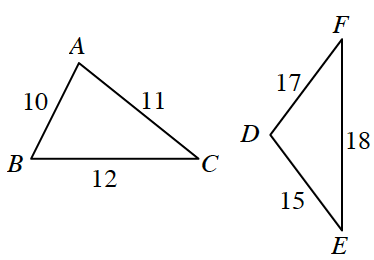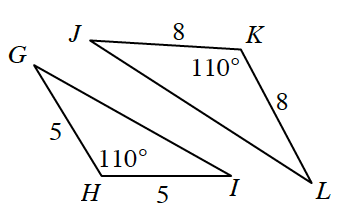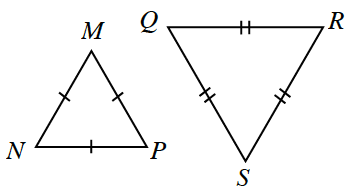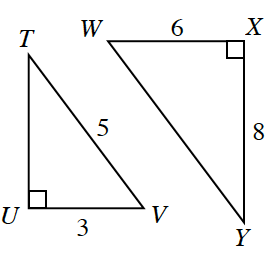### Home > INT2 > Chapter 9 > Lesson 9.1.1 > Problem9-11

9-11.

Decide if the triangles in each pair below are similar. If they are similar, give the similarity condition and a sequence of transformations that maps the left triangle onto the right triangle. If they are not similar, explain how you know.

1.not similar

1.$SAS \sim$; Possible sequence: Translate $ΔGHI$ so that $H'$ lies on point $K$, rotate about $H'$ so that the angle sides coincide, dilate by a scale factor of $8/5$.

1.These are both equilateral triangles.

1.